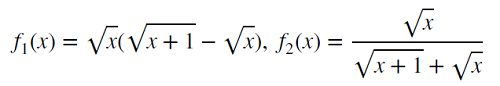# Computer error and programmer mistakes in MATLAB programming

49

## Description

MATLAB number ONE introduces one of the best course in here. This course is related to mistakes that most MATLAB user can use in their code.

This is course is a general course and can be useful for all programmer that program with other programming languages such as Java, C, C++, Python, C#, ASP.NET.

You can not find this course in other MATLAB references such as help documentation.

If you want to understand the importance of this course, consider these examples :

```» x=2ˆ30;

» x+2ˆ-22==x

ans= 0(false)

» x+2ˆ-23==x```
```ans= 1(true)

```

The result is amazing, when you add a number with 2^(-23), the final value is equal with the number. What does happen in here? You will know after learning this course.

For another example, consider the following two formulas:For mathematical viewpoints, these equations are the same, but after writing a simple code, we see that the final value are different when you increase x. Why these two equations generate different results? Perhaps, we could not trust the final result of our code.

Do you know, what is bad subtraction? In this course, you will learn about it.

## Some topics in this course are :

IEEE 64-bit Floating-Point Number Representation.

Truncation error.

Round-off error.

Overflow/underflow.

Loss of significance.

Error magnification.

Absolute/Relative Computing Errors.

Error Propagation.

Tips for Avoiding Large Errors.

Nested Computing for Computational Efficiency.

Vector Operation or Loop Iteration.

To Avoid Runtime Error.

All programmer

Scantiest

Developer

MATLAB users

## Reviews

There are no reviews yet.

SKU: COMERRHUMIS21 Categories: ,